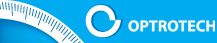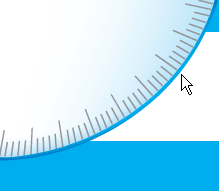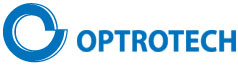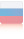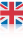OPTICAL DIGITAL MEASURING SYSTEMSCOMPANYPRODUCTSRESEARCHSERVICEPUBLICATIONPATENTSACHIEVEMENTPARTNERSCONTACTSRESEARCH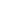Automated optical digital linearity deviation meterNew concept of angle measurement; Simulation and experimental studiesLinear and engular measurements using CCD camerasPrecision of image positioning by CCD cameraDigital Two-Dimensional Autocollimator with resolution 0.001” of engleCalibration of Digital Two-Dimensional AutocollimatorMetrological research and selection of target shape in digital measuring systemsLinewidth Measurement in Optical and Atomic-force Microscope for Metrological Control of PhotomasksMeasurement of diameter and geometry of precision ball bearingsMeasurement and correction of distortion in coordinate measurements by CCD cameraRESEARCH# Digital Two-Dimensional Autocollimator Calibration

The device calibration was conducted using precise engle measuring table (AMT) with the flat mirror mounted on its rotary module. The root-mean-square error of measurements with the mirror significantly exceeds the error when the BKR-180º prism is used. Therefore for calibration and measuring purposes the method of combined measurements with the evaluation of linear regression was used. This method allows to reduce the effect of random errors, caused by the mechanical instability of the test bench.

The comparative analysis of AMT and DAC concludes that their metrological parameters of engle coordinate measurements are sufficiently close. The resolution of both devices equals to 0.001''.

Two cycles of measurements were conducted for each axis (X, Y).

Table 4 shows the results of the first cycle of engle measurement during rotation of the mirror, mounted on the engle measuring table (AMT), for axis X. The readings measurement renge for axis Y was not more than 0.5''of engle.

Table 4

 # engle Measuring Table(engular seconds) DAC Х(engular seconds) Trend Estimate (engular seconds) 3-4(engular seconds) 1 2 3 4 5 1 49.42 515.454 515.569 -0.115 2 100.12 464.532 464.462 0.070 3 151.00 413.220 413.173 0.047 4 200.45 363.301 363.326 -0.025 5 251.64 311.647 311.725 -0.078 6 300.48 262.512 262.493 0.019 7 350.96 211.549 211.608 -0.059 8 414.31 147.830 147.749 0.081 9 451.82 109.988 109.938 0.050 10 500.53 60.845 60.837 0.008 11 551.86 9.208 9.094 0.114 12 601.20 -40.673 -40.642 -0.031 13 650.85 -90.696 -90.690 -0.006 14 700.30 -140.562 -140.538 -0.024 15 751.97 -192.651 -192.622 -0.029 16 801.79 -242.839 -242.843 0.004 17 855.49 -296.949 -296.974 0.025 18 901.36 -343.199 -343.212 0.013 19 952.11 -394.397 -394.370 -0.027 20 1000.37 -442.939 -443.017 0.078 21 1052.05 -495.222 -495.112 -0.110 СКО 0.061

Fig.1 shows the linear regression based on the combination of readings of AMT and DAC (columns 2, 3) for cycle X1. The coefficient for the linear regression term represents an estimated value.

Fig. 1.
Linear regression based on combination of readings of AMT and DAC for cycle X1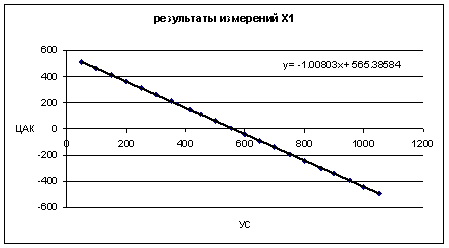Column 4 in Table 4 shows the estimates based on the regression formula х = -1.00803x + 565.38584, and column 5 – the difference between the DAC real measurements and the formula estimates, where x are readings of AMT. Values in column 5 can be considered as a total error of calibration, determined by the metrological parameters of both devices. In the bottom of the table MSE of the total error, equal to 0.061'', is given. Fig. 2 shows the total error for X1 cycle.

Fig. 2 Total error for X1 cycle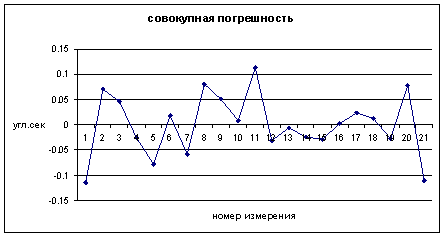For the second cycle of measurements (X2) we derived the following regression formula: y = -1.00797x + 565.42955, where x indicates the readings of the goniometrical table (AMT), and MSE of the total error equals 0.049''. We assume the value of the calibration coefficient for axis X as the mean value of the two cycles - (1.00803+1.00799)/2 = 1.00801. MSE of the total error 0.05'' and the renge of the total error of 0.1'' for two devices with a similar accuracy is a good indication of high quality of the measurements. For the calibration on axis Y the autocollimator tube was rotated 90 degrees, while the measurement renge for axis X did not exceed 0.5''.
Table 5 shows the results of the first cycle of the engle measurement for axis Y, with the AMT mirror rotated.

Table 5

 # engle Measuring Table(engular seconds) DAC Х(engular seconds) Trend Estimate(engular seconds) 3 – 4(engular seconds) 1 2 3 4 5 1 200.56 312.419 312.407 0.012 2 250.69 262.009 261.879 0.130 3 300.52 211.616 211.652 -0.036 4 352.11 159.669 159.652 0.017 5 407.67 103.597 103.651 -0.054 6 453.17 57.670 57.789 -0.119 7 501.29 9.221 9.286 -0.065 8 552.24 -42.092 -42.069 -0.023 9 600.71 -90.897 -90.924 0.027 10 652.26 -142.957 -142.884 -0.073 11 702.68 -193.504 -193.705 0.201 12 800.52 -292.360 -292.323 -0.037 СКО 0.089

Fig.3 shows the linear regression based on the combination of readings of AMT and DAC for cycle Y1.

Fig. 3.
Linear regression based on combination of readings of AMT and DAC for cycle Y1.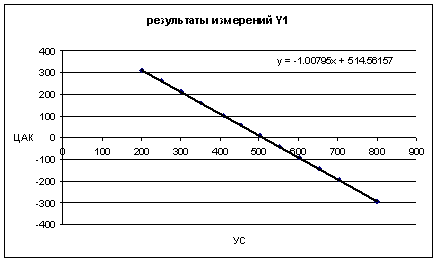Column 4 of Table 5 shows the estimates based on the regression formula y = -1.00795x + 514.56157, and column 5 – the difference between the DAC real measurements and the formula estimates, where x are readings of AMT. Values in column 5 can be considered as a total error of calibration, determined by the metrological parameters of both devices. In the bottom of the table MSE of the total error, equal to 0.061'', is given. Fig. 4 shows the total error for Y1 cycle.

Fig. 4 Total error for Y1 cycle.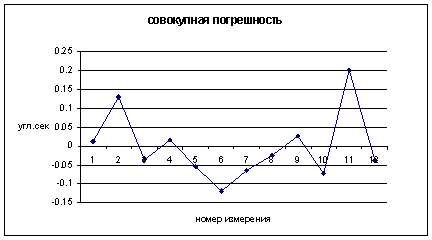For the second cycle of measurements (Y2) we derived the following regression formula: y = -1.00813x + 514.71177, where x indicates the readings of the goniometrical table (AMT), and MSE of the total error was equal to 0.055''. We assume the value of the calibration coefficient for axis Y as the mean value of the two cycles - (1.00795+1.00813)/2 = 1.00804. Proximity of the calibration coefficients, RMS of the total error equal to 0.05'' and renge of the total error less than 0.1'' for both axes is also a good indication of the high measurement quality.

Follow-up Measurements

Follow-uo measurements were performed after the calibration coefficients were entered into the program. Since AMT is not certified for engle measurements, follow-up measurements were also conducted based on the regression analysis of the combined measurement results.

Table 6 shows the results of the follow-up engle measurements with the AMT mirror rotated toward axis X.

Fig.5 shows the linear regression curve based on combination of the readings of AMT and DAC (columns 2, 3) for axis X measurements.

Table 6

 # engle Measuring Table(engular seconds) DAC Х(engular seconds) Trend Estimate(engular seconds) 3 – 4(engular seconds) 1 2 3 4 5 1 50.76 505.622 505.695 -0.073 2 152.03 404.442 404.417 0.025 3 252.61 303.814 303.829 -0.015 4 353.65 202.767 202.781 -0.014 5 452.41 104.042 104.013 0.029 6 552.48 4.001 3.935 0.066 7 652.21 -95.771 -95.803 0.032 8 750.85 -194.377 -194.451 0.074 9 852.23 -295.891 -295.839 -0.052 10 951.99 -395.656 -395.607 -0.049 11 1052.00 -495.655 -495.625 -0.030 СКО 0.049

Column 4 of Table 6 shows the estimates based on the regression formula y = -1.00008x + 556.71177, and column 5 – the difference between the DAC real measurements and the formula estimates, where x are readings of AMT. Values in column 5 can be considered as a total error of calibration, determined by the metrological parameters of both devices. In the bottom of the table RMS of the total error, equal to 0.049", is given. Fig. 5 shows the total error for X axis measurements.

Fig. 5 Combined error for measurements in X direction plot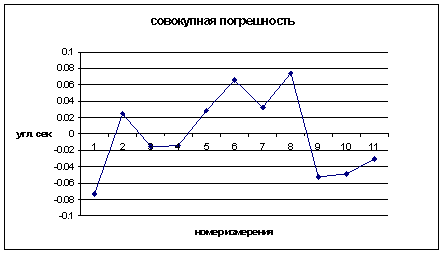For combined Y axis measurements we derived the following regression formula: y = -0.9999x + 510.56146, where x are readings of goniometrical table and column 5 shows the difference between real measurements and the formula estimates, whereas and RMS of the total error was equal to 0.048''.

Conclusion.

The results of above research work and measurements enable us to draw the following conclusions:

1. In accordance with the linear regression formulas for follow-up measurements, the coefficients for the linear regression term differ from 1 within ± 0.0001. It means that the calibration error on the limit of the controlled renge ± 8' does not exceed 0.05'' and for the renge ± 1' – no more than 0.06''.

2. For both axes RMS of the total error does not exceed 0.05''.

3. For both axes the maximum spread of the total error does not exceed 0.08''.

4. As a result, the total error of digital autocollimators is within 0.1" (it should be noted that this is just the upper metrological limit of the device accuracy, referenced to the measurement tool, i.e. the engle measurement table)

Top